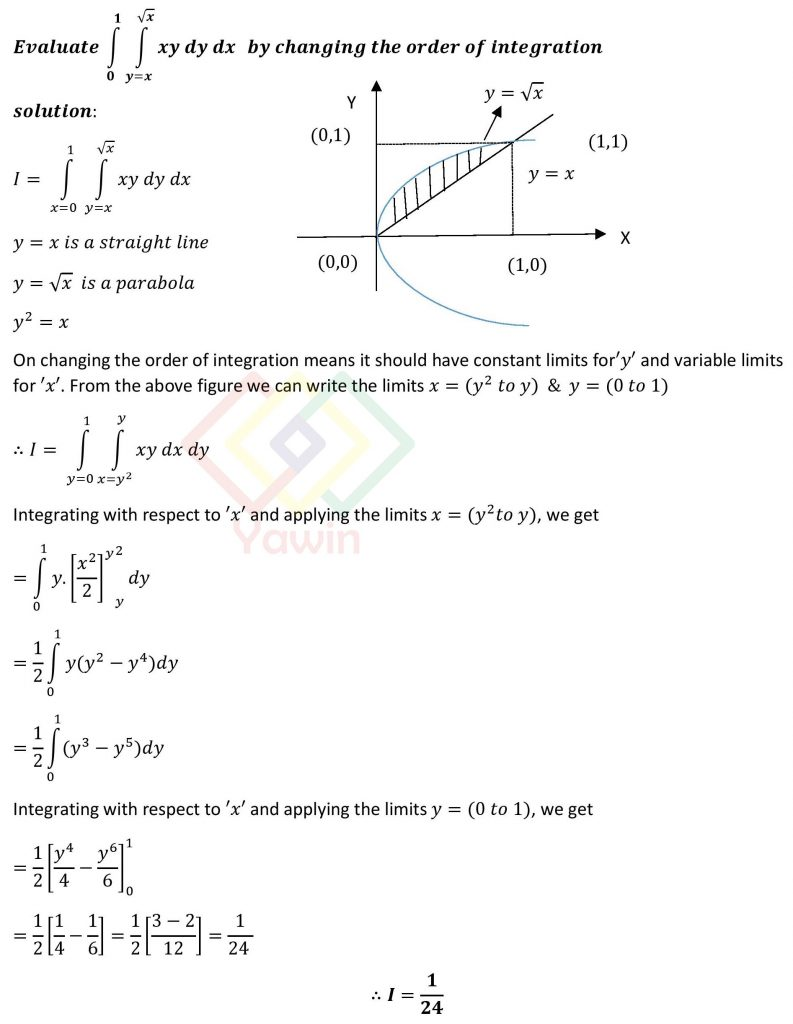Evaluate double integral of xy dy dx over the limits x=(0 to 1) and y=(x to sqrt(x)) by changing the order of integration

## Question## Question

Evaluate double integral of xy dy dx over the limits x=(0 to 1) and y=(x to sqrt(x)) by changing the order of integration

## Topic

Types of Evaluation of Double Integral